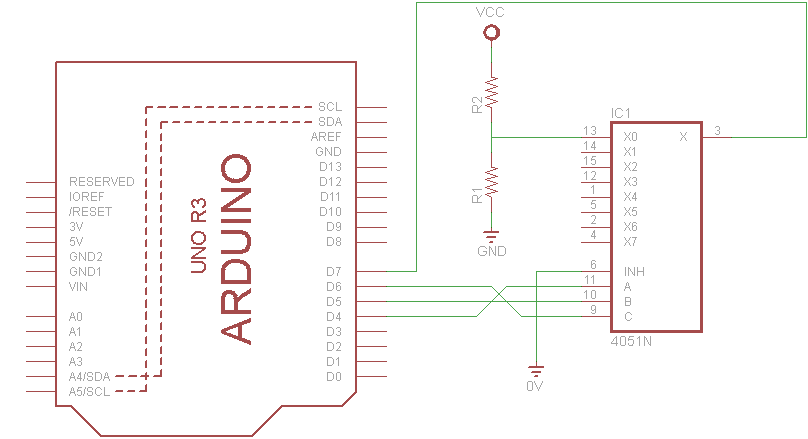Home LearningBasics Arduino and 4051 LED example

# Arduino and 4051 LED example

In previous posts we showed some examples for the 4067 16-Channel multiplexer – Arduino and 4067 LED example

The HCF4051 device is a monolithic integrated circuit fabricated in MOS (metal oxide semiconductor) technology available in SO-16 and PDIP-16 packages.

The HCF4051 analog multiplexer/demultiplexer is a digitally controlled analog switch having low ON impedance and very low OFF leakage current. This multiplexer circuit dissipates extremely low quiescent power over the full VDD- VSSand VDD- VEEsupply voltage range, independent of the logic state of the control signals.

This device is a single 8-channel multiplexer having three binary control inputs, A, B, and C, and an inhibit input. The three binary signals select 1 of 8 channels to be turned on, and connect one of the 8 inputs to the output. When a logic “1” is present at the inhibit input terminal all channels are off.

Here is a pinout of the 4051

Lets look at the truth table of the 4051 which will show how this works

Now lets have an example, we want to flash an LED on Channel AO which is Pin 13 of the IC, in this case A0, A1 and A2 all have to be a logic 0. So with our micro we can make the pins that we connect to A0, A1 and A2 all low and that will activate channel A0. In our example we have the following wiring

A0 – Arduino D4
A1  – Arduino D5
A2 – Arduino D6

INH or Enable is tied to 0v

In the schematic below we show 1 resistor and LED only, our test board had 8 sets of resistors and LEDsCode

The code is actually adapted from the 4067 example, I saw a couple of whacky code examples which to be honest i couldn’t get working. This example will cycle through all 8 channels one at a time.

 Source code```const int channel[] = {4, 5, 6};

//the output pin - mux input
const int outputPin = 7;

void setup()
{
// set up all pins as output
for (int arduinoPin = 4; arduinoPin <= 7; arduinoPin++)
{
pinMode(arduinoPin, OUTPUT);
}
}

void loop()
{
//iterate through all 8 channels of the multiplexer
for (int muxChannel = 0; muxChannel < 8; muxChannel++)
{
//set the channel pins based on the channel you want
//LED on - high - 1000 milliseconds delay
muxWrite(muxChannel);
digitalWrite(outputPin,HIGH);
delay(1000);
}
}

void muxWrite(int whichChannel)
{
for (int inputPin = 0; inputPin < 3; inputPin++)
{